A cyclic process ABCD is shown in the P-V diagram. Which of the following P-T curves represent the same process ?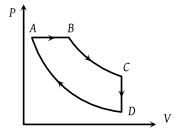(1)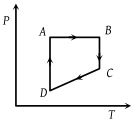(2)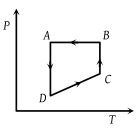(3)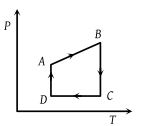(4)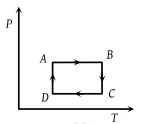Concept Questions :-

Types of processes
High Yielding Test Series + Question Bank - NEET 2020

Difficulty Level:

Carnot cycle (reversible) of a gas represented by a Pressure-Volume curve is shown in the diagram . Consider the following statements

I. Area ABCD = Work done on the gas

II. Area ABCD = Net heat absorbed

III. Change in the internal energy in cycle = 0

Which of these are correct ?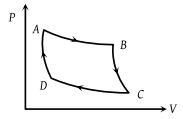(1) I only

(2) II only

(3) II and III

(4) I, II and III

Concept Questions :-

First law of thermodynamics
High Yielding Test Series + Question Bank - NEET 2020

Difficulty Level:

The temperature-entropy diagram of a reversible engine cycle is given in the figure. Its efficiency is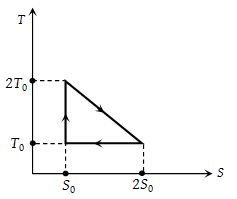(1) 1/3

(2) 2/3

(3) 1/2

(4) 1/4

Concept Questions :-

Heat engine and refrigerator
High Yielding Test Series + Question Bank - NEET 2020

Difficulty Level:

Work done in the given P-V diagram in the cyclic process is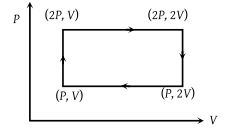(1) PV

(2) 2PV

(3) PV/2

(4) 3PV

Concept Questions :-

Work done by gas
High Yielding Test Series + Question Bank - NEET 2020

Difficulty Level:

A cyclic process ABCA is shown in the V-T diagram. Process on the P-V diagram is -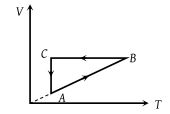(1)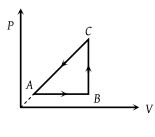(2)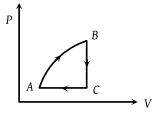(3)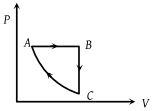(4)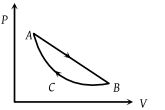Concept Questions :-

Types of processes
High Yielding Test Series + Question Bank - NEET 2020

Difficulty Level:

In the figure given two processes A and B are shown by which a thermo-dynamical system goes from initial to final state F. If ΔQA and ΔQB are respectively the heats supplied to the systems then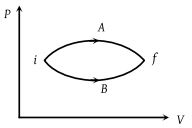(1) ΔQA = ΔQB

(2) $\Delta {Q}_{A}\ge \Delta {Q}_{B}$

(3) ΔQA < ΔQB

(4) ΔQA > ΔQB

Concept Questions :-

First law of thermodynamics
High Yielding Test Series + Question Bank - NEET 2020

Difficulty Level:

In the cyclic process shown in the figure, the work done by the gas in one cycle is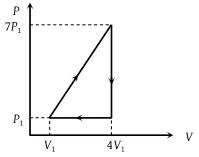(1) 28 P1V1

(2) 14 P1V1

(3) 18 P1V1

(4) 9 P1V1

Concept Questions :-

Work done by gas
High Yielding Test Series + Question Bank - NEET 2020

Difficulty Level:

Heat energy absorbed by a system in going through a cyclic process shown in figure is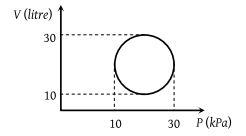(1) 107 π J

(2) 104 π J

(3) 102π J

(4) 10–3π J

Concept Questions :-

Cyclic process
High Yielding Test Series + Question Bank - NEET 2020

Difficulty Level:

A thermodynamic system is taken from state A to B along ACB and is brought back to A along BDA as shown in the PV diagram. The net work done during the complete cycle is given by the area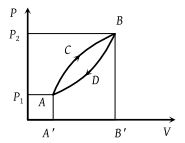(1) P1ACBP2P1

(2) ACBB'A'A

(3) ACBDA

Concept Questions :-

Work done by gas
High Yielding Test Series + Question Bank - NEET 2020

Difficulty Level:

In the diagrams (i) to (iv) , variation of volume with changing pressure is shown. A gas is taken along the path ABCD. The change in internal energy of the gas will be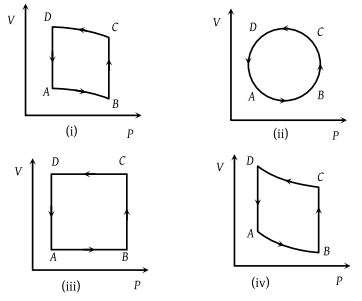(1) Positive in all cases (i) to (iv)

(2) Positive in cases (i), (ii) and (iii) but zero in (iv) case

(3) Negative in cases (i), (ii) and (iii) but zero in (iv) case

(4) Zero in all four cases

Concept Questions :-

Cyclic process